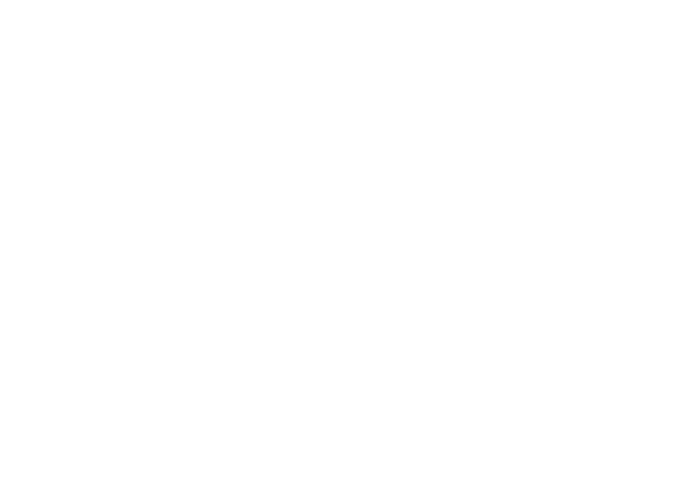# Integers and natural numbers: which are there more of?

Mariama is a 7th grade student who loves math. She told us in a letter she wrote to Sam, the main character of our middle school narrative. What she wrote amazed us, let’s see what you think.

I have a question that I think you might be able to help me answer. What do you think there is more of, natural numbers or integers? I would also like to know why.

I perfectly remember the feeling I had when I read the letter, particularly, when I read the question. My eyes filled with tears and I started shouting euphorically around the office because I felt the need to share that letter with everyone. Every time I read it I get goose bumps. In a way, it was a small piece of evidence that teachers, and stories like Sam’s Journey, can bring about the change we want to see in our students. We can help them ask the questions they need to ask to grow, and guide them to discover the answers.

A twelve-year-old girl was asking herself a question that was crucially relevant in the history of mathematics. And now, we were faced with a great challenge, how do we respond to this letter. As the great German mathematician Georg Cantor said,

This blog post was inspired by Mariama and so many other students like her. Inspired by what they are able to come up with when they are given the chance to express themselves.

## The importance of the question

At first glance, the answer to Mariama’s question may seem obvious. If we ask ourselves how many natural numbers there are, the answer that comes to mind is ‘infinite’. The same is true if we ask how many integers there are, so it may seem obvious to answer: “There are the same amount, infinite.” But somehow, it seems that there have to be more integers than naturals, right? After all, natural numbers make up only part of the set of integers. If we leave zero aside, each natural number has another opposite integer (1, -1; 2, -2; etc.). Therefore, we are missing something.

Infinity is an extremely complex and not very intuitive concept, but at the same time it is fascinating. The documentary A trip to Infinity, directed by Jonathan Halperin and Drew Takahashi, shows this very well. In fact, it was not until the 19th century that Georg Cantor was able to begin to shed light on all this darkness. Interested in the study of infinity, Cantor initiated what is known as set theory, which has been (and is) essential for the development of mathematics. In the words of another great German mathematician, David Hilbert:

Hilbert was also very interested in infinity and was one of the staunch defenders of Cantor’s theory, which during that time had many detractors because of the highly revolutionary ideas that were inherent to it. With that theory, Cantor was able to formalize that there are infinities of different sizes. But what about integers and natural numbers? Is their infinity the same size or not? In order to understand the most elementary ideas that Cantor formalized about infinity, we should travel back in time a few thousand years.

## The root of it all: counting

The first hints of mathematics arise from the need to count. Now we have it easy. To count, we use numbers. But in the old days they did not use numbers as we know them today….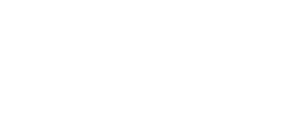If a shepherd wanted to keep track of the number of sheep in the flock, he only had to have as many stones as sheep. If he wanted to put the sheep out to pasture, he could keep track of how many left the farm by setting aside a stone for each sheep that had left. And then return the stones one by one, as the sheep returned.

In this way, the most fundamental concept of counting consists of comparing sets of elements by establishing a 1-to-1 relationship. By establishing the 1-to-1 relationship between two sets, A and B, for example, we can match each element of A with a single element of B, and if there are elements from B left over, then the number of elements of B is greater. In fact, a child who does not know how to count will probably follow this strategy to compare two sets.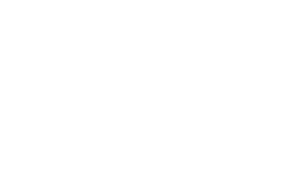“There are more yellow cubes than green cubes because there is one yellow cube left unpaired with a green cube.”

If, instead, we can establish a 1-to-1 correspondence between the elements of the sets, that is, a 1-to-1 correspondence for all the elements of both sets, we know that the two sets have the same number of elements.

## Integers vs. natural numbers

In that case, what happens when we want to compare sets with an infinite number of elements, such as integers and naturals? We follow this same idea of comparing elements 1-to-1: we say that two sets are equally large if we can establish a 1-to-1 correspondence between these two sets. Let’s consider the set of natural numbers and the set of integers:

ℕ={0, 1, 2, 3…}       ℤ={…, -3, -2 -1, 0, 1, 2, 3…}

Perhaps, at first glance, it seems that we cannot establish this correspondence, but if we think a little, we see that we can associate, in an orderly way, the non-negative integers to the even natural numbers, and the negative integers to the odd natural numbers: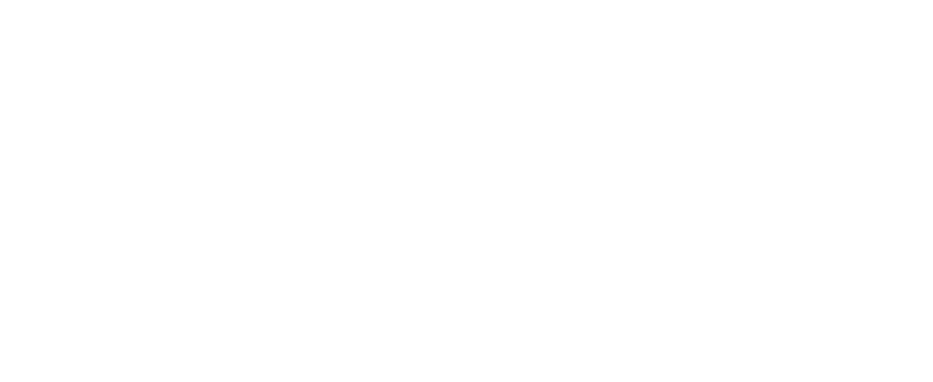As this correspondence is 1-to-1, we can say that the number of integers is the same as the number of natural numbers. As Cantor formalized, the infinity of the naturals is just as large as the infinity of the integers. And this opens up a fascinating and extremely complex world to investigate, full of very interesting questions that we will not go into now. But if you are interested in exploring infinities of different sizes and being able to analyze other sets such as rational numbers, or the interval [0.1], you can read the magnificent article ‘How Big Is Infinity?’, in Quanta Magazine.

That said, it would seem that the number of integers and natural numbers is the same. And the same would be true if we were to compare the number of even numbers with the number of natural numbers. But there’s something that doesn’t quite convince us, isn’t there? Intuitively, we would still think that there are half as many even numbers as natural numbers, just as there are one-fifth as many multiples of 5 as natural numbers. Well, perhaps we should change our point of view to find a way in which mathematics can answer intuition.

## Formalizing intuition

When we move away from these set-theoretic infinities and move into number theory, natural density appears in the mathematical literature, a concept that attempts to describe how large a subset of numbers is within the set of natural numbers. This concept of density is closely linked to probabilistic ideas and fits very well with what we intuitively think, but it also has some drawbacks, which we will not go into today.

We imagine that we want to analyze, for different values of 𝑛, the proportion of even numbers smaller than 𝑛 within the set of natural numbers smaller than 𝑛. If we call P(𝑛) the number of even numbers less than 𝑛, and study the ratio P(𝑛) / 𝑛 for different values of 𝑛, we get: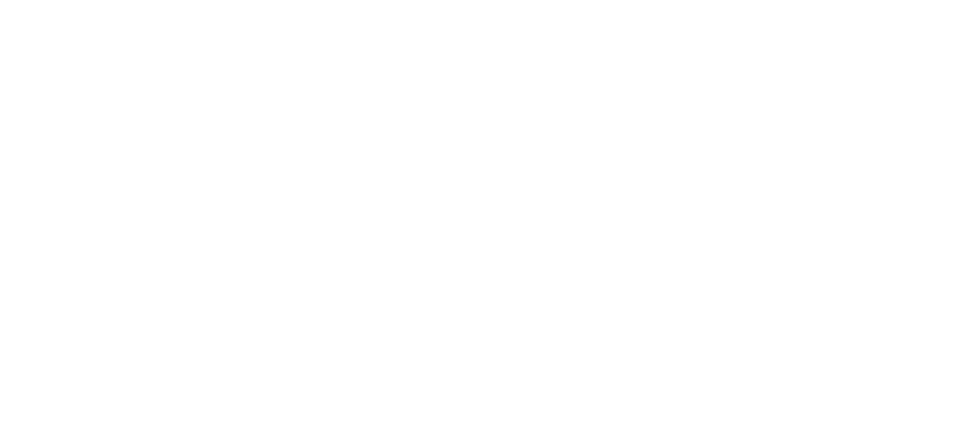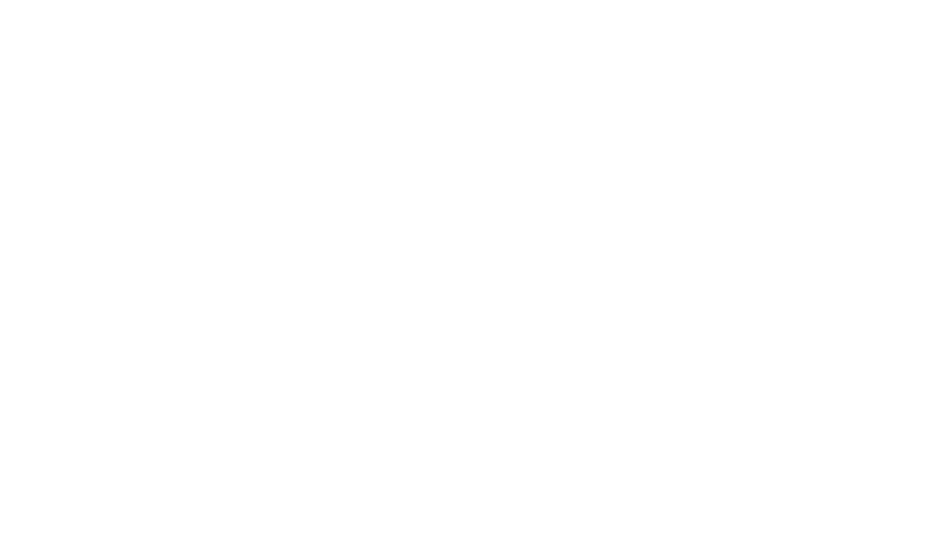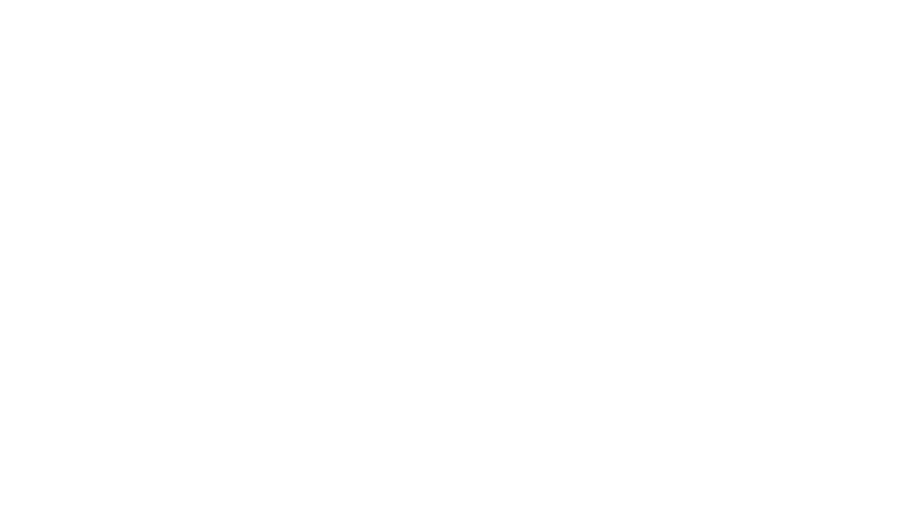As we can see, as we increase 𝑛, the ratio of the number of even numbers less than 𝑛 to the number of natural numbers less than 𝑛 gets as close as we want to 1/2.

With this background idea, of going to the limit when 𝑛 tends to infinity, the natural density of even numbers, which equals 1/2, is defined. This density can be extended, although not always, to other subsets of the natural numbers.
But Mariama was not asking about subsets of natural numbers; she wanted to compare integers with natural numbers. Although a similar concept to integer density is not very widespread in the literature, we could construct it in a similar way, taking the set of integers as a reference instead of the set of natural numbers. We call Z(𝑛) the number of integers of absolute value less than 𝑛. For example, for 𝑛 = 10, we have:

Z(10) = #{-9, -8, -7, -6, -5, -4, -3, -2, -1, 0, 1, 2, 3, 4, 5, 6, 7, 8, 9} = 19

If we want to study the proportion of natural numbers less than 𝑛 within the set of integers of absolute value less than 𝑛, we can replicate the reasoning we followed earlier to compare even numbers and natural numbers: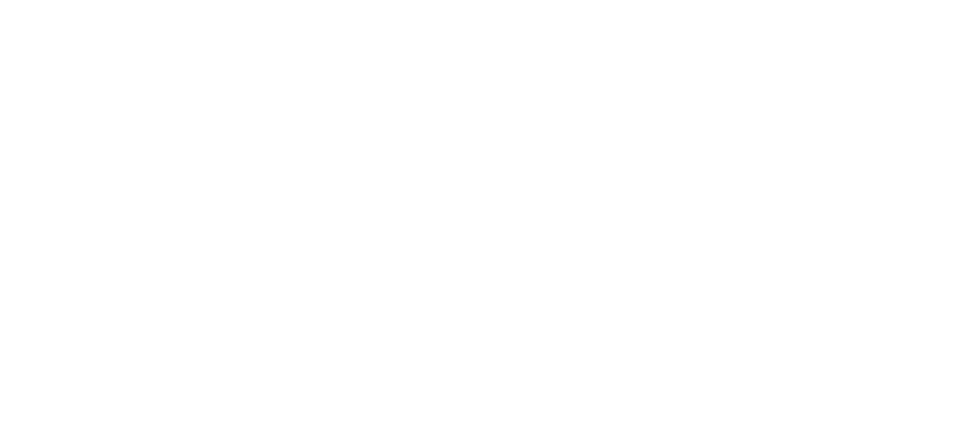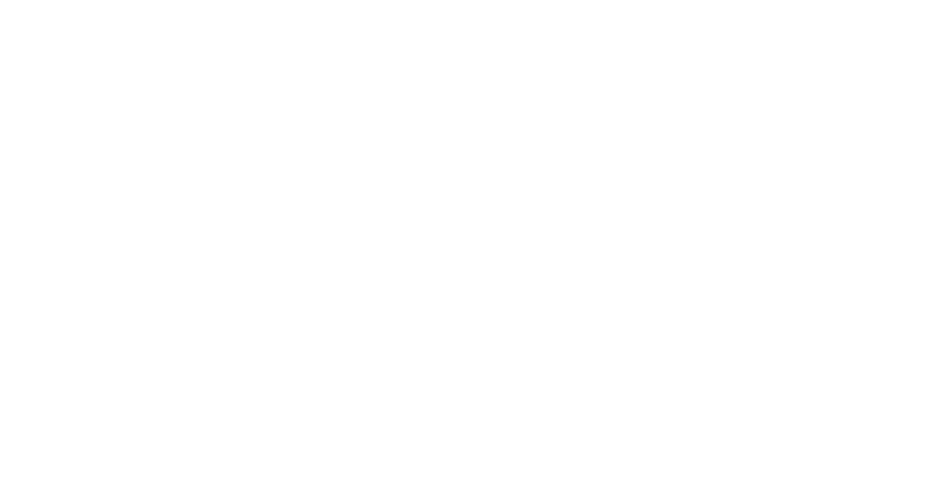As before, we can also see that, as we increase 𝑛, the quotient of the number of natural numbers less than 𝑛 by the number of absolute-valued integers less than 𝑛 gets as close as we want to 1/2.
If we again go to the limit when 𝑛 tends to infinity, we can define the integer density of natural numbers, which is equal to 1/2.

These ideas of densities of subsets in the set of natural numbers have aroused the interest of many important mathematicians, such as the Hungarian Paul Erdös (1913-1996), considered one of the most prolific mathematicians of all time; or the Australian Terrence Tao, one of the most important mathematicians of today. And there are still many unknowns to be discovered in this topic.

## And the conclusions?

So, although it is true that we can say, thanks to Cantor, that the set of integers is equally as large as the set of natural numbers, we also have probabilistic arguments to say that there are half as many natural numbers as integers. Perhaps you feel disappointed for not having a categorical answer to the question asked, or perhaps on the contrary, you feel satisfied to discover that mathematics is a much more complex and creative world than you imagined and that there is not always a single answer to a question.

In fact, there is no branch of mathematics that does not have unanswered questions; questions that the mathematical community has asked (and continues to ask) make this discipline advance and grow tirelessly.

Let us not be afraid of our students asking questions in the classroom that we do not know how to answer; on the contrary, let us welcome them and value them greatly. Let’s show them that mathematics is a world that is enriched by questions that we are eager to find answers to. Hopefully we can help Mariama, driven by this curiosity, to continue asking questions that will contribute her grain of sand to the mathematical community.

### Do you want to take this to the classroom?

In Session 14: "In search of 0" In the first year of middle school, we worked on the students' ability to ask themselves questions.

•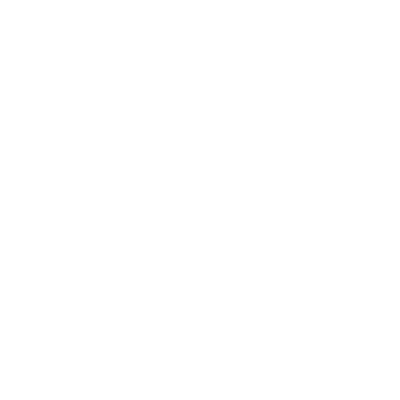Trained Engineer and a vocational Mathematics Teacher. Currently he is a math teacher in Sant Gregori school. He combines his teaching work with teacher training and content creation in the Innovamat’s Education department.

# Recent posts